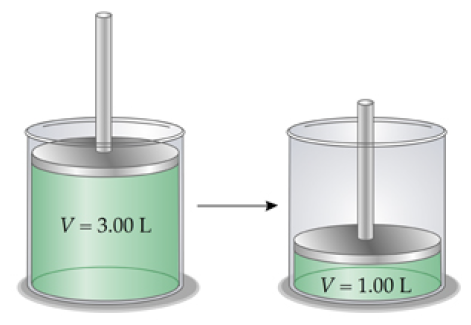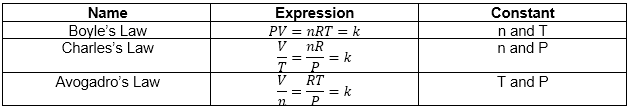# Problem: A certain amount of chlorine gas was placed inside a cylinder with a movable piston at one end. The initial volume was 3.00 L and the initial pressure of chlorine was 1.95 atm. The piston was pushed down to change the volume to 1.00 L. Calculate the final pressure of the gas if the temperature and number of moles of chlorine remain constant.Express your answer with the appropriate units.The ideal gas law (PV = nRT) describes the relationship among pressure P, volume V, temperature T, and molar amount n. When some of these variables are constant, the ideal gas law can be rearranged in different ways to take the following forms where k is a constant:

###### FREE Expert Solution
86% (107 ratings)View Complete Written Solution
###### Problem Details

A certain amount of chlorine gas was placed inside a cylinder with a movable piston at one end. The initial volume was 3.00 L and the initial pressure of chlorine was 1.95 atm. The piston was pushed down to change the volume to 1.00 L. Calculate the final pressure of the gas if the temperature and number of moles of chlorine remain constant.The ideal gas law (PV = nRT) describes the relationship among pressure P, volume V, temperature T, and molar amount n. When some of these variables are constant, the ideal gas law can be rearranged in different ways to take the following forms where k is a constant: